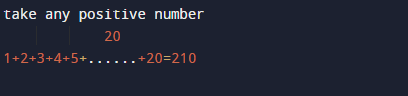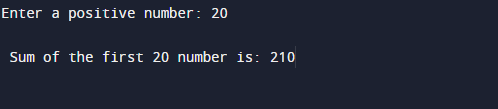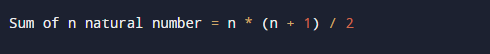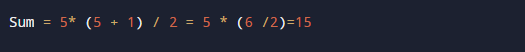C program to calculate sum of n numbers

In this tutorial you will learn about the C program to calculate sum of n numbers and its application with practical example.

In this tutorial, we will learn to create a C++ program that will calculate the sum of n natural number using C++ programming.

Prerequisites

Before starting with this tutorial we assume that you are best aware of the following C programming topics:

• C Operators.
• C loop statements.

Logic behind to Calculate the Sum of Natural Numbers.

All natural numbers contains  positive value  starting from 1, 2, 3, to n numbers . let us take an example to calculate the sum of starting 20 numbers. Here  we start adding the numbers from 1 to the given number suppose 20 is a given , this process is called the sum of N natural numbers.

ExampleC program to calculate sum of n numbers.

In this program we will calculate sum of n numbers with use of loop.
Firstly we declare required header file and variable and also initiates required values in variable. Next we take value from user at run time and then after we will  add the given number values.

OutputMathematical Explanation

Here we represent to find the sum of n natural numbers using the mathematical formula:If we want to calculate the sum of the first 5 natural number using above formula.In our program, we have first declared and initialized a set variables required in the program.

• no = number for calculating n natural number.
• sum= adding all natural numbers.
• i= i for loop iteration .

In the above program, when user enter a positive number 20 and stores it in variable no the loop continuously iterates over and over till it reaches condition where  i <=20. In every  iteration, the value of i is added to sum, and i is incremented by 1 in every loop. When the condition false, it exits the loop and prints the sum of 20 natural numbers.

In this tutorial we have learn about the C program to calculate sum of n numbers and its application with practical example. I hope you will like this tutorial.